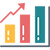### Cost Accounting

#### Concept and Calculation of Mark Up

###### Content ContributorsPriya KaurChristian Bien

# Learning Objectives###### ​###### What is Mark-Up?Mark up is the percentage or amount added to the price or quote in the production of goods or services to cover costs and produce a profit. Businesses have a purpose of producing the largest profit available, without a markup, businesses will be running at breakeven levels producing no profit. In other words, it's the % of gross profit on a sale or providing a service.

For example, if a painter was asked for a quote for a job and the job cost \$100 but he quoted the customer \$110, then the markup would be 10% or \$10.###### Calculation of a Mark-UpTo calculate a markup simply multiply the cost of a manufacture of a product or delivery of a service by the percentage amount.

Example 1: If a job cost \$150. A 20% markup would be: = \$150 * 1.20 = \$180

Example 2: If a job cost \$200 A 15 % markup would be: = \$200 * 1.15 = \$230

Example 3: If a job cost \$500 A 45% markup would be: =\$500 *1.45 =\$725###### Finding the Percentage of Mark-UpWhile this is unlikely to show up in a test or exam, to find the percentage markup remember: (New -Old)/Old * 100

Example 1: If a quote was \$725 and the cost of the job is \$500. The markup would be: = (725-500)/500 *100 =225/500 *100 = 0.45 *100 =45%

Example 2: If a quote was \$800 and the cost of the job is \$600 The markup would be: =(800-600)/600 *100 =200/600 *100 =0.33 *100 =33%###### ​###### ​###### ​###### ​###### ​